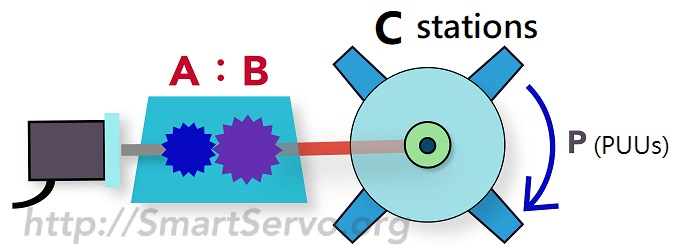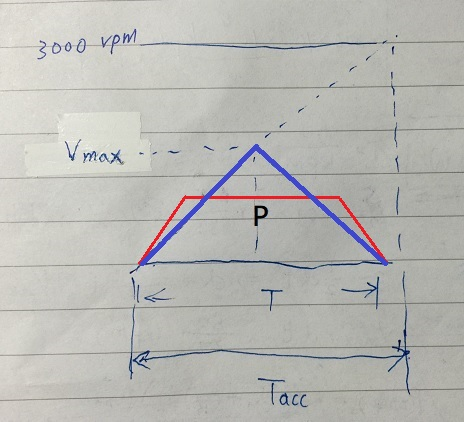# Electronic gear ratio (on-line) calculator – Indexer/Turret

This article provides an online calculator for the Indexer/ATC turret/Flying shear rotary cut mechanism to quickly find the servo’s electronic gear ratio and provide additional simulation information to evaluate whether the system parameters meet the requirements. The steps are as follows:

1. Enter the number(C) of Tools or Stations.
2. Define Division, ie, How many PUUs betweens 2 stations.
3. the Circumference(C×P) is displayed and which is used for P2-52 of Delta A2 servo.
4. Enter the mechanical Reduction ratio (1:1 without deceleration)
5. Enter Encoder Resolution(PLS), PLS number per turn of the Encoder,ie,The number of PUUs required to make the motor rotate 1 turn when the electronic gear ratio is 1:1.
6. Press the “Calculate” button to get the numerator and denominator of the E-Gear Ratio.
7. Enter the positioning time T of each division to check whether the simulation result is satisfied?﻿

Input Data： Enter the number(C) of Tools/Stations = ie,the division number.

Define 1 division：P =  PUU（Pos of User Unit）

Circumference：C × P = PUU => for P2-52 of Delta Servo Drive

1st Reduction ratio：A1 =   ： B1 = A1 < B1 for deceleration

2nd Reduction ratio：A2 =   ： B2 = A2 < B2 for deceleration

3rd Reduction ratio：A3 =   ： B3 = A3 < B3 for deceleration

Encoder's Resolution = （PLS/Rev）PLS number per turn of the Encoder

Result：Electronic Gear Ratio　 Num = ：Den =
Simulation infomation：=> Use triangle speed profile

1 station positioning time T ＝ 　（ms），means command duration

Results： Acc/DeAcc Time(Tacc) ＝   ms，form 0 ~ 3000 rpm

Max Command speed(Vmax)＝   rpm，Must <= the motor's max speed

Max Pulse Freq. ＝   kpps ( KPUU/Sec), Must < PLC's output capability

PUUs per turn of motor ＝  PUU/Rev ，recommended 　＞　5000

formula：circumference(C x P) x Num／Den = R(PLS/Rev) x B1B2B3／A1A2A3

The simulation information will be displayed when the positioning time(T) of a division is entered. The description of each field is as follows :

1. Acceleration/deceleration time(Tacc) : If the acceleration/deceleration time of the controller is set to Tacc calculated by the tool, the positioning time of one division is exactly equal to the specified T. If the set value > Tacc, the positioning time exceeds T; otherwise, if the set value < Tacc, the positioning time is less than T. Tacc represents the time it takes for the motor speeds up from 0 to 3000 rpm. The smaller the value, the faster the acceleration !
2. Maximum Command speed(Vmax) : should not exceed the maximum speed of the motor. If it occurs, reduce the reduction ratio or increase the positioning time T. It can also reduce the controller’s acceleration and deceleration time to reduce the maximum speed.
3. Maximun Pulse Frequency : Must be less than the highest output pulse frequency of the controller (PLC), otherwise the required line speed cannot be reached. If it is exceeded, please modify the PUU unit to increase the electronic gear ratio ! This message can be ignored if the command comes from the drive itself (eg Delta ASD-A2 Smart Servo)
4. PUUs per turn of motor : It is recommended that this value not be less than 5000 to avoid jitter when the motor rotates. The lower the speed, the more obvious! Reducing the electronic gear ratio can improve this.

The triangle speed profile and symbol definition are shown in the blue line below, where the acceleration and deceleration are symmetrical:The reason why the triangular velocity profile is selected is that the acceleration and deceleration of the triangular curve is softer (compared to the trapezoid) at a given positioning distance P and time T, but the maximum speed is higher,Therefore, as long as the maximum speed Vmax does not exceed the motor limit, setting the controller’s acceleration/deceleration time to the Tacc calculated by the tool can satisfy the specified positioning time with a softer triangular curve! However, if the maximum speed Vmax exceeds the motor limit, if you do not want to increase the positioning time T, you must reduce the acceleration/deceleration time to reduce the maximum speed. As shown in the red trapezoid curve above, the cost is that the acceleration/deceleration slope will be steeper !

Ref: E-Gear ratio calculator –  for Belt/Roller mechanism .